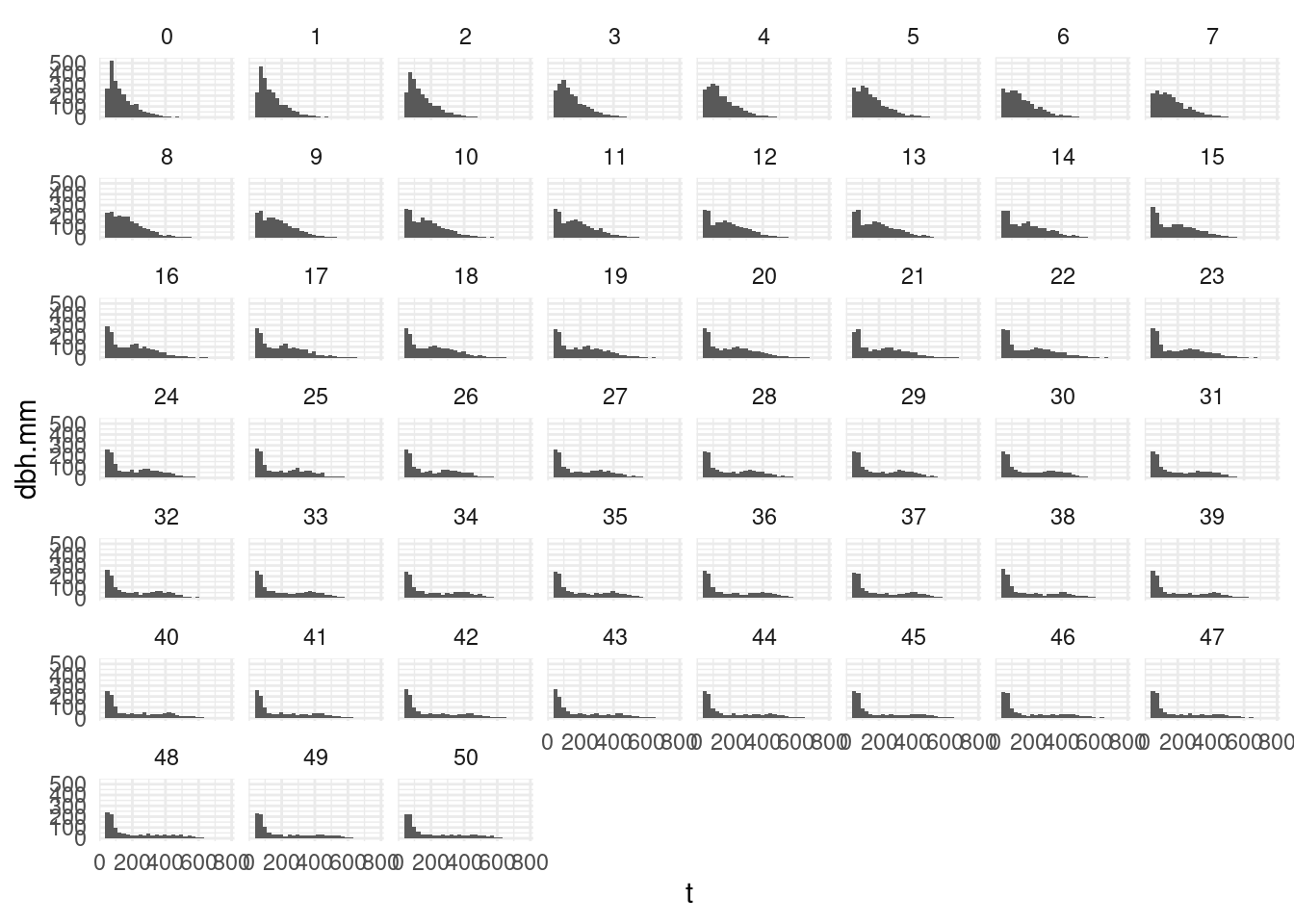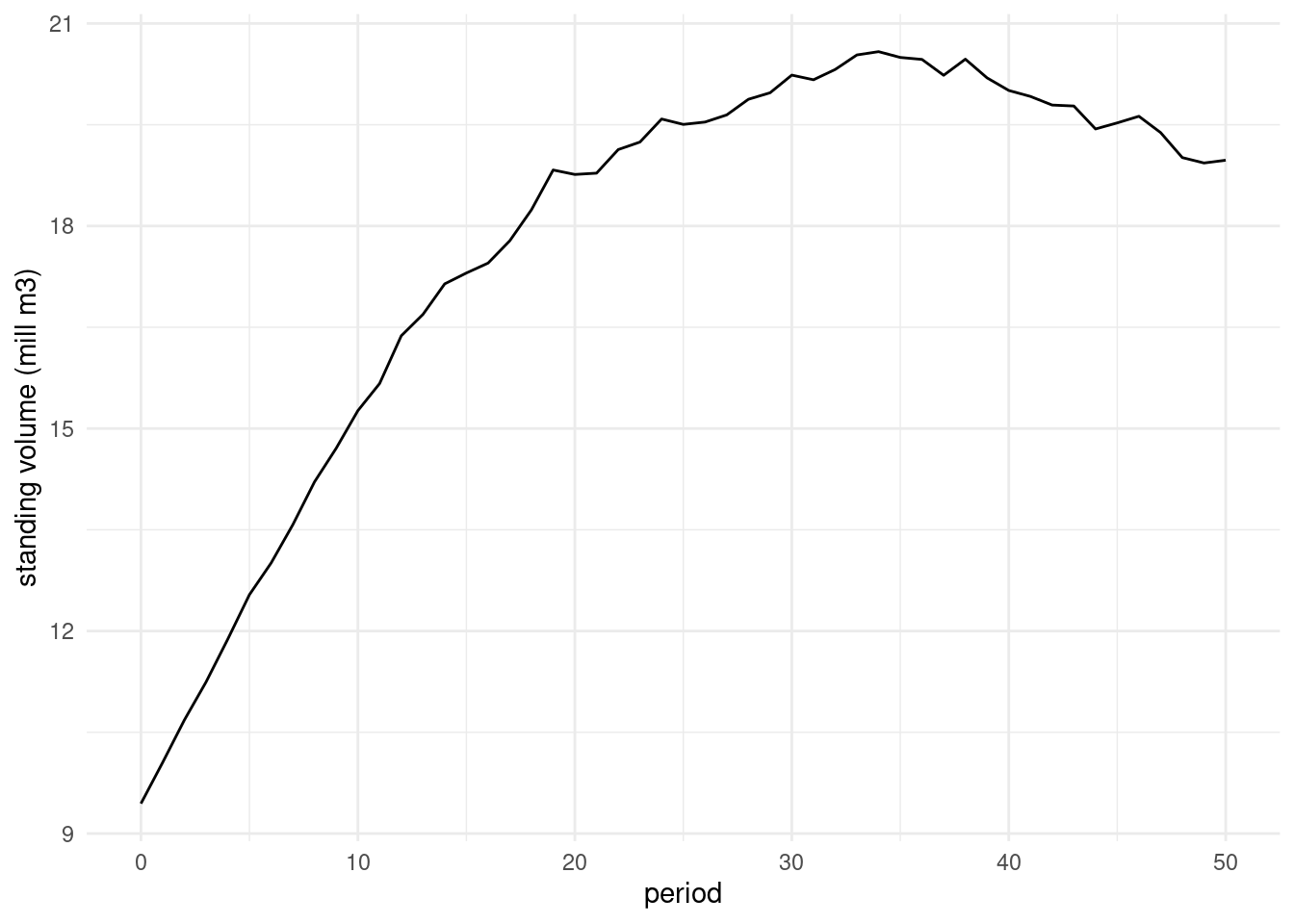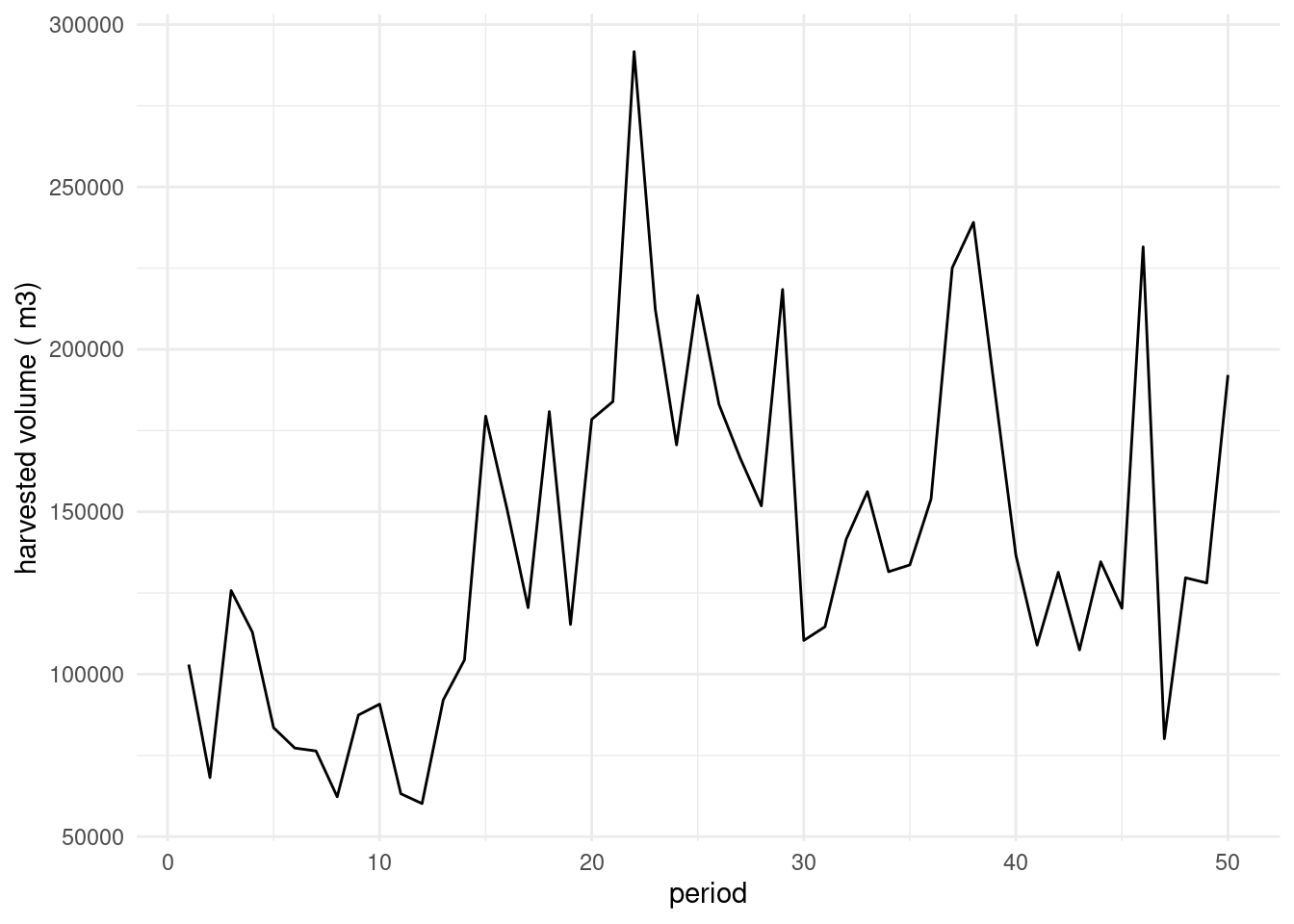# Introduction

This tutorial gives examples on how you can use sitree to simulate individual tree growth, death, and harvest through time, and to compare the effects of changing functions (e.g. use a different mortality function) in the results of the simulation.

For a general introduction and description of the package take a look at the ‘Introduction to SiTree’ vignette.

# A little note on Reference classes

SiTree uses reference classes to keep memory usage at bay. Bare in mind that reference classes such as trList and trListDead are modified in place. For example,

``````## Always bare in mind that RF trList and trListDead are modified in place
## Do not copy

Polygon <- setRefClass("Polygon", fields = c("sides"))
square <- Polygon\$new(sides = 4)

square.to.triangle <- function(a){
## This makes the object triangle point to square, it does not create a copy
## since square is a RC object
triangle <- square
## when we modifiy triangle we are modifying square.
triangle\$sides <- 3
return(a+2)
}

## square has 4 sides
square\$sides``````
``##  4``
``````## when we call the square.to.triangle function the 'square' object
## gets modified, eventhough we don't pass it as argument. That is because
## it is referenced inside the function
square.to.triangle(2)``````
``##  4``
``square\$sides``
``##  3``
``````## but if we do
square <- Polygon\$new(sides = 4)

square.to.triangle <- function(a){
triangle <- square\$copy()
triangle\$sides <- 3
return(a+2)
}

square\$sides``````
``##  4``
``square.to.triangle(2)``
``##  4``
``````## the object remains unchanged
square\$sides``````
``##  4``

Most regular users will never need to use these methods.

Let's see now some examples of the methods implemented for these RF.

``````library(sitree)
res <- sitree (tree.df   = tr,
stand.df  = fl,
functions = list(
fn.growth     = 'grow.dbhinc.hgtinc',
fn.mort       = 'mort.B2007',
fn.recr       = 'recr.BBG2008',
fn.management = 'management.prob',
fn.tree.removal = 'mng.tree.removal',
fn.modif      = NULL,
fn.prep.common.vars = 'prep.common.vars.fun'
),
n.periods = 5,
period.length = 5,
mng.options = NA,
fn.dbh.inc = "dbhi.BN2009",
fn.hgt.inc =  "height.korf",
fun.final.felling = "harv.prob",
fun.thinning      = "thin.prob",
per.vol.harv = 0.83
)

## getTrees(i, j)  -- obtains the information of the i trees, on the j periods,
## by default it selects all. It does not display it, it passes the value.
## It returns a list with elements plot.id, treeid, dbh.mm, height.dm, yrs.sim,
## tree.sp

get.some.trees <- res\$live\$getTrees(1:3, 2:5)

## extractTrees(i)  -- extracts the i trees, it removed the trees from the
## original object and it passes the information. It returns a list.

## addTrees(x) -- x should be a list

## last.time.alive. It checks when was the last DBH measured.
last.measurement = cbind(
, args = list(
growth = data.frame(dbh.inc.mm     = rep(3, 4),
hgt.inc.dm  = rep(8, 4)),
mort   = rep(TRUE, 4),
this.period = "t2")
),
),
nperiods = res\$live\$nperiods
)

## which in this case differs from the data stored under the last.measurement
## field because we have defined it artificially above as "t3"
lta``````
``````##    6    7    8    9
## "t5" "t5" "t5" "t5"``````
`` new.dead.trees\$last.measurement\$found.dead``
``##  "t3" "t3" "t3" "t3"``
`````` ## But we can remove the data from the periods after it was found dead
## and now results do match
## last time it was alive was in "t2"
lta``````
``````##    6    7    8    9
## "t2" "t2" "t2" "t2"``````
`````` ## ant it was found dead in "t3"
``##  "t3" "t3" "t3" "t3"``

# Taking a look at the results of the simulation

We first use the example functions available in the sitree package to run a 15 periods simulation.

``````set.seed(2017)

n.periods <- 50
res <- sitree (tree.df   = tr,
stand.df  = fl,
functions = list(
fn.growth     = 'grow.dbhinc.hgtinc',
fn.mort       = 'mort.B2007',
fn.recr       = 'recr.BBG2008',
fn.management = 'management.prob',
fn.tree.removal = 'mng.tree.removal',
fn.modif      = NULL,
fn.prep.common.vars = 'prep.common.vars.fun'
),
n.periods = n.periods,
period.length = 5,
mng.options = NA,
fn.dbh.inc = "dbhi.BN2009",
fn.hgt.inc =  "height.korf",
fun.final.felling = "harv.prob",
fun.thinning      = "thin.prob",
per.vol.harv = 0.83
)``````

## dbh distribution through time

``````dbh.mm <- res\$live\$data\$dbh.mm
dbh.mm.short <- reshape(dbh.mm,
varying = paste0("t", 0:n.periods),
timevar = "period",
direction = "long", sep = "")
``````##     period   t id
## 1.0      0 234  1
## 2.0      0 173  2
## 3.0      0  86  3
## 4.0      0 230  4
## 5.0      0 236  5
## 6.0      0 234  6``````
``````dbh.mm.short\$t[dbh.mm.short\$t == 0] <- NA
library(ggplot2)
ggplot(dbh.mm.short, aes(x = t)) + geom_histogram() + ylab('dbh.mm') +
facet_wrap(~ period) + theme_minimal()``````
``## `stat_bin()` using `bins = 30`. Pick better value with `binwidth`.``
``## Warning: Removed 255723 rows containing non-finite values (stat_bin).``## Volume distribution through time

And since we already have the code to calculate volume we can easily plot the evolution of standing volume or harvested volume

``````vol <- data.frame(matrix(NA, ncol = n.periods+1, nrow = length(res\$plot.data\$plot.id)))
names(vol) <- paste0("t", 0:n.periods)
for (i.period in 0:n.periods){
sa <- prep.common.vars.fun (
tr = res\$live, fl= res\$plot.data,
i.period, this.period = paste0("t", i.period),
common.vars = NULL,
period.length = 5 )

vol[, (i.period +1)] <- sa\$res\$vuprha.m3.ha
## This is volume per ha, if we prefer just m3 we multiply by ha2total
## ha2total is the number of ha represented by the plot
vol[, (i.period +1)] <- vol[, (i.period +1)] * sa\$fl\$ha2total
}
vol.m3.short <- reshape(vol,
varying = paste0("t", 0:n.periods),
timevar = "period",
direction = "long",
sep = "")
vol.m3.short\$t[vol.m3.short\$t == 0] <- NA
total.standing.volume <- aggregate(t ~ period, data = vol.m3.short, FUN = sum)
total.standing.volume\$vol.mill.m3  <- total.standing.volume\$t/1e6

ggplot(total.standing.volume,
aes(period, vol.mill.m3))   + geom_line()+
ylab("standing volume (mill m3)") + theme_minimal()``````## Harvested volume

``````vol <- data.frame(matrix(NA, ncol = n.periods + 1,
nrow = length(res\$plot.data\$plot.id)))
## res\$removed\$data only contains the "history of the tree", but we need
## the dbh and height of the tree at harvest time
names(vol) <- paste0("t", 0:n.periods)
removed <- recover.last.measurement(res\$removed)

for (i.period in 0:n.periods){
harv.vol <- prep.common.vars.fun (
tr = res\$removed,
fl = res\$plot.data,
i.period,
this.period = paste0("t", i.period),
common.vars = NULL,
period.length = 5 )

vol[, (i.period +1)] <- harv.vol\$res\$vuprha.m3.ha
## This is volume per ha, if we prefer just m3.
vol[, (i.period +1)] <- vol[, (i.period +1)] * sa\$fl\$ha2total
}
names(vol) <- paste0(substr(names(vol), 1, 1), ".", substr(names(vol), 2, 3))

vol.m3.short <- reshape(vol,
varying = paste0("t.", 0:n.periods),
timevar = "period",
idvar = "id",
direction = "long"
)
vol.m3.short\$t[vol.m3.short\$t == 0] <- NA
harv.total <- aggregate(t ~ period, data = vol.m3.short, FUN = sum)

ggplot(harv.total, aes(period, t))   + geom_line()+
ylab("harvested volume ( m3)") + theme_minimal()````````````vol <- data.frame(matrix(NA, ncol = n.periods + 1,
nrow = length(res\$plot.data\$plot.id)))
names(vol) <- paste0("t", 0:n.periods)

for (i.period in 0:n.periods){
vol[, (i.period +1)] <-
prep.common.vars.fun (
fl= res\$plot.data,
i.period,
this.period = paste0("t", i.period),
common.vars = NULL,
period.length = 5 )\$res\$vuprha.m3.ha

## This is volume per ha, if we prefer just m3.
vol[, (i.period +1)] <- vol[, (i.period +1)] * sa\$fl\$ha2total
}

names(vol) <- paste0(substr(names(vol), 1, 1), ".", substr(names(vol), 2, 3))
vol.m3.short <- reshape(vol,
varying = paste0("t.", 0:n.periods),
timevar = "period",
idvar = "id",
direction = "long"
)

``````##     period t id
``````## let's plot dead trees by plot, for the first 10 plots